### Geometry II

What is the combined measure of one orange and one blue angle (as marked below)?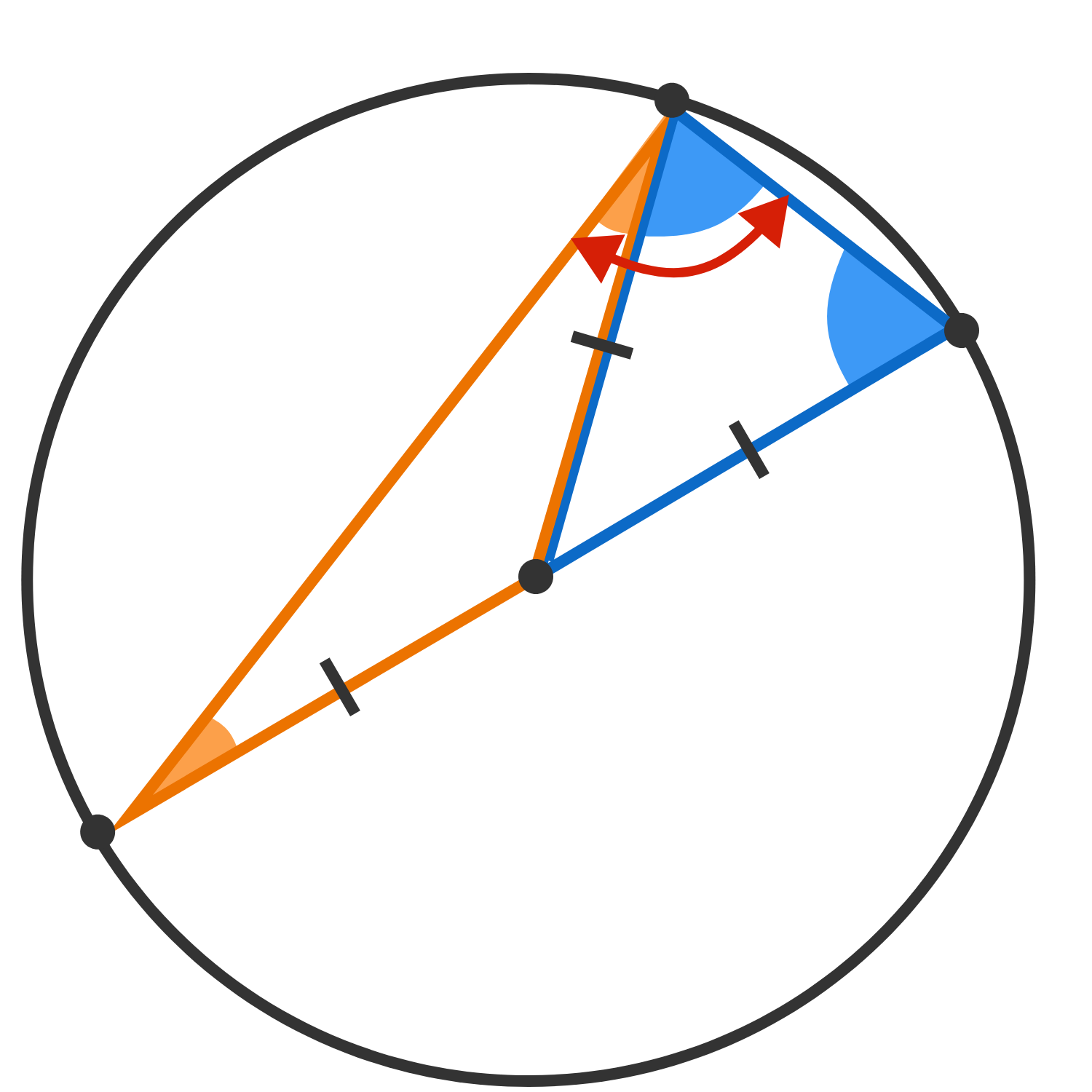(Note: The line passing through the centre is a diameter).

# Thales' Theorem

Thales' theorem states that if a triangle has all three vertices on the circumference of a circle and the diameter of the circle as one of its sides, then the triangle will always be a right triangle.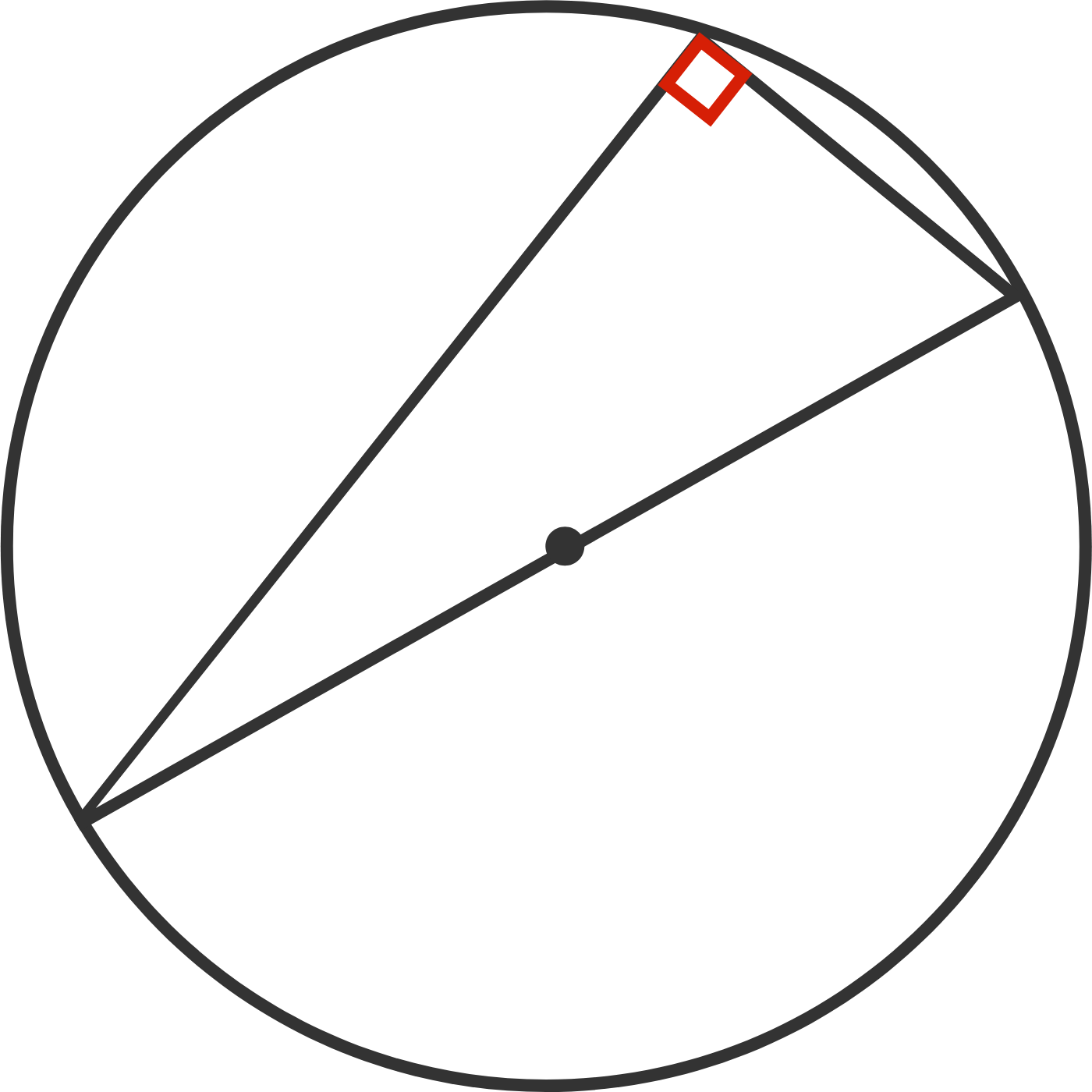In the diagram below, we have a triangle with three vertices on the circumference that has one side as the diameter of the circle. We created another triangle by connecting one vertex of the triangle to the circle's center.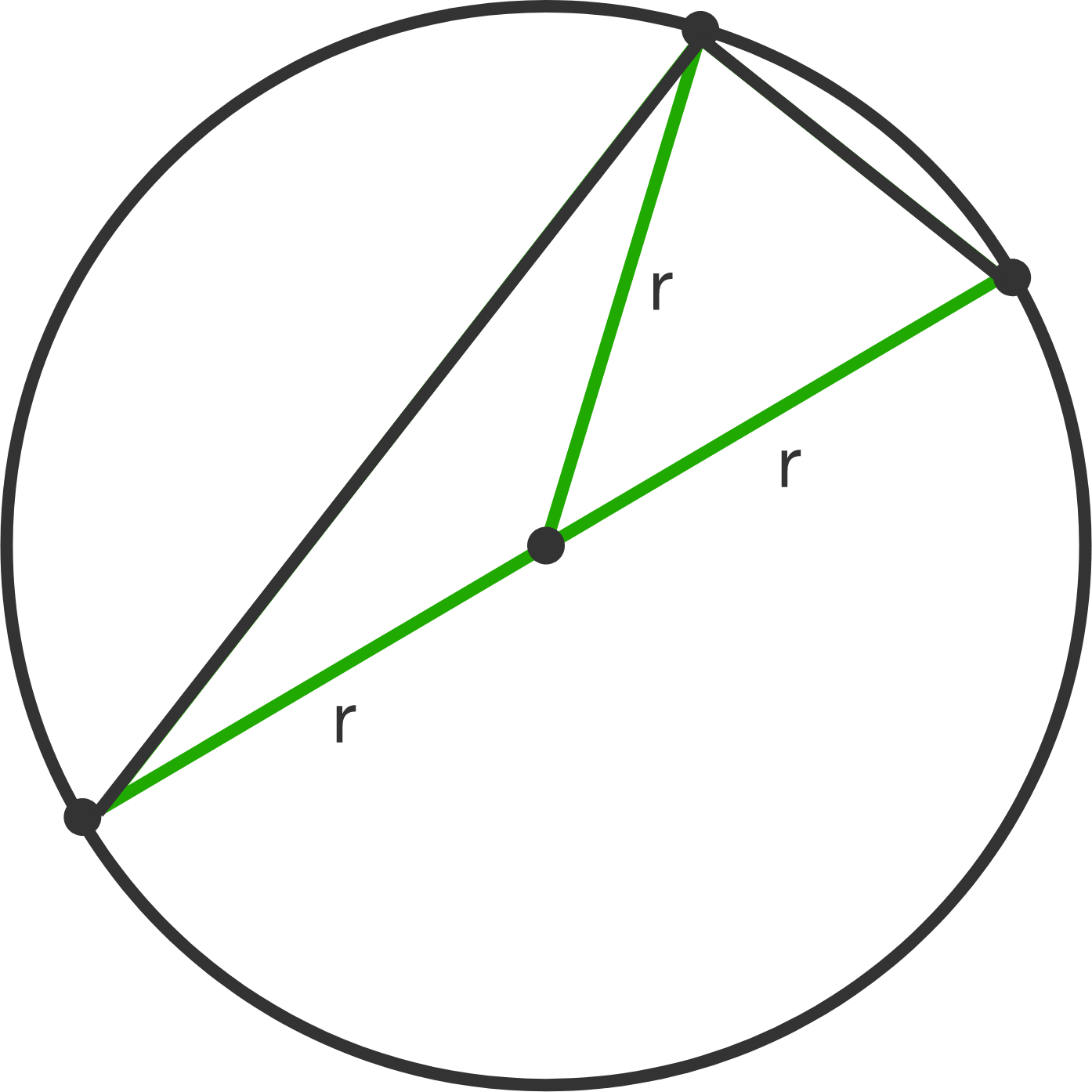Since each radius has identical length $r,$ we have two isosceles triangles, one shown in blue and one shown in orange.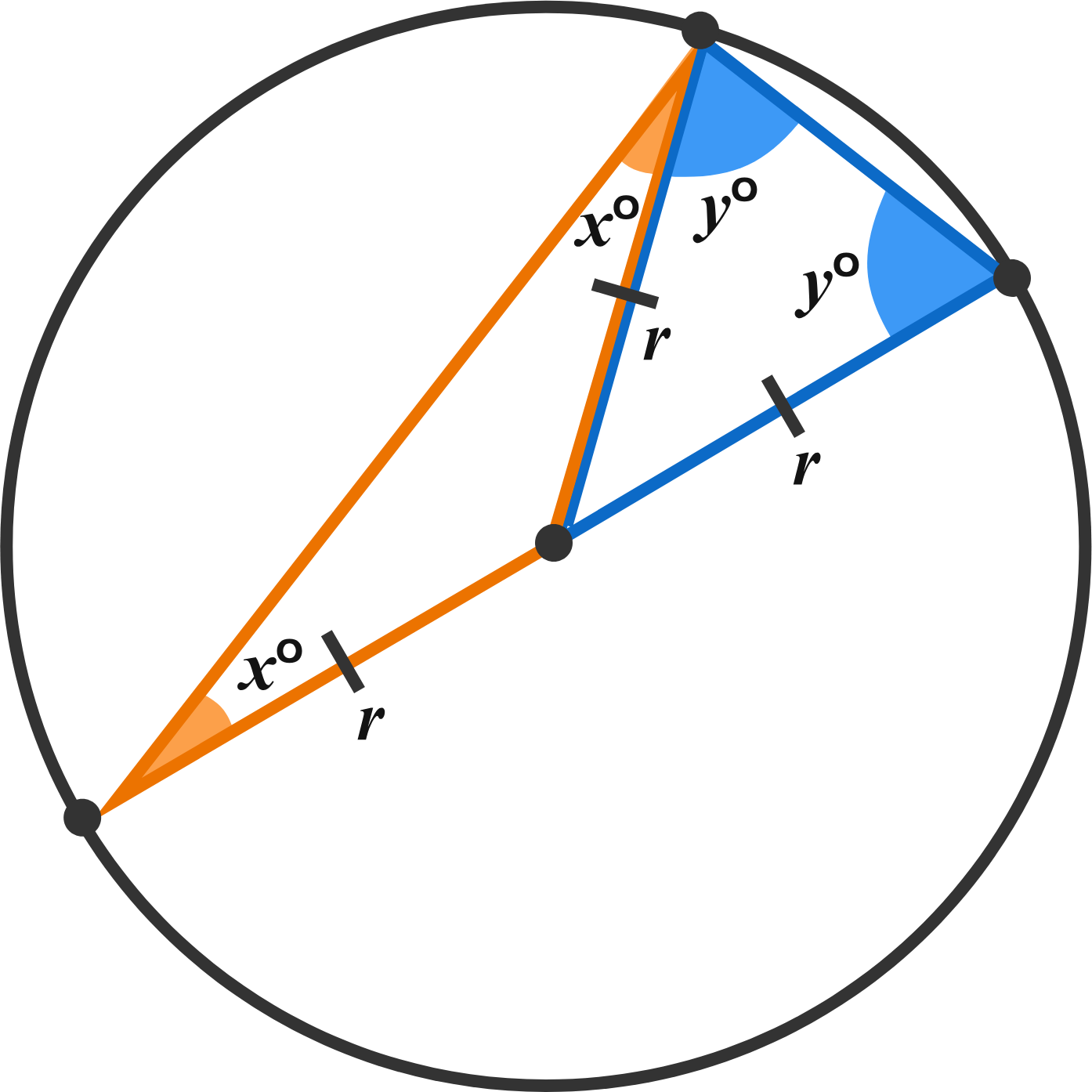Because they form the base angles in an isosceles triangle, the two orange angles are congruent and the two blue angles are congruent. Writing an equation to represent the three angles in the original, we know that \begin{aligned} x + y + x + y &= 180^\circ \\ 2x + 2y &= 180^\circ \\ x + y &= 90^\circ .\end{aligned}

# Thales' Theorem

If $\overline{CD}$ is the diameter of the circle, which is larger: the measure of $\angle A$ or the measure of $\angle B \, ?$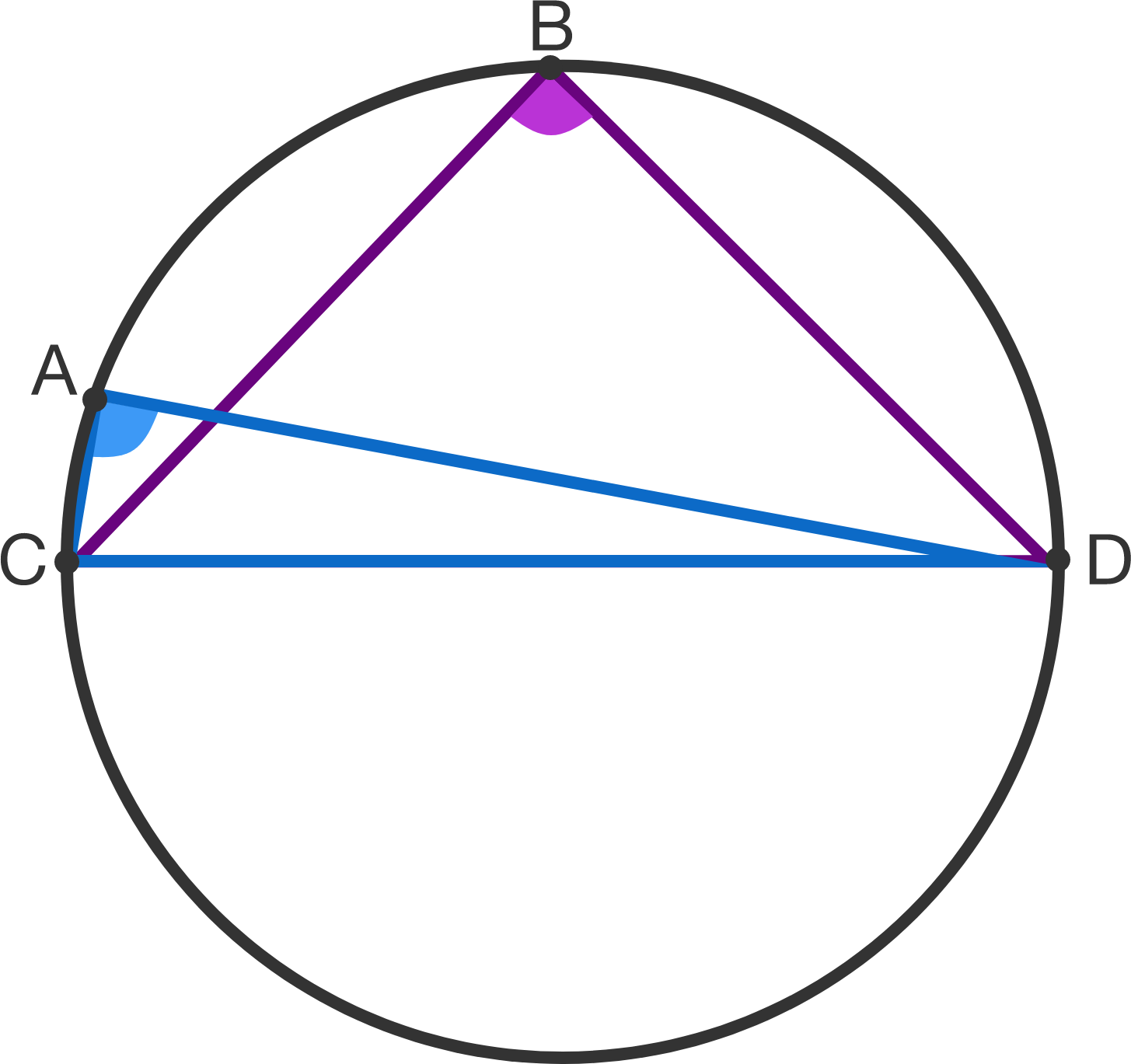Note: $A$ and $B$ both lie on the circle.

# Thales' Theorem

Now we'll test if the theorem works both ways.

True or false?

If a right triangle is inscribed in a circle, then its hypotenuse must be a diameter of the circle that passes through the circle's center.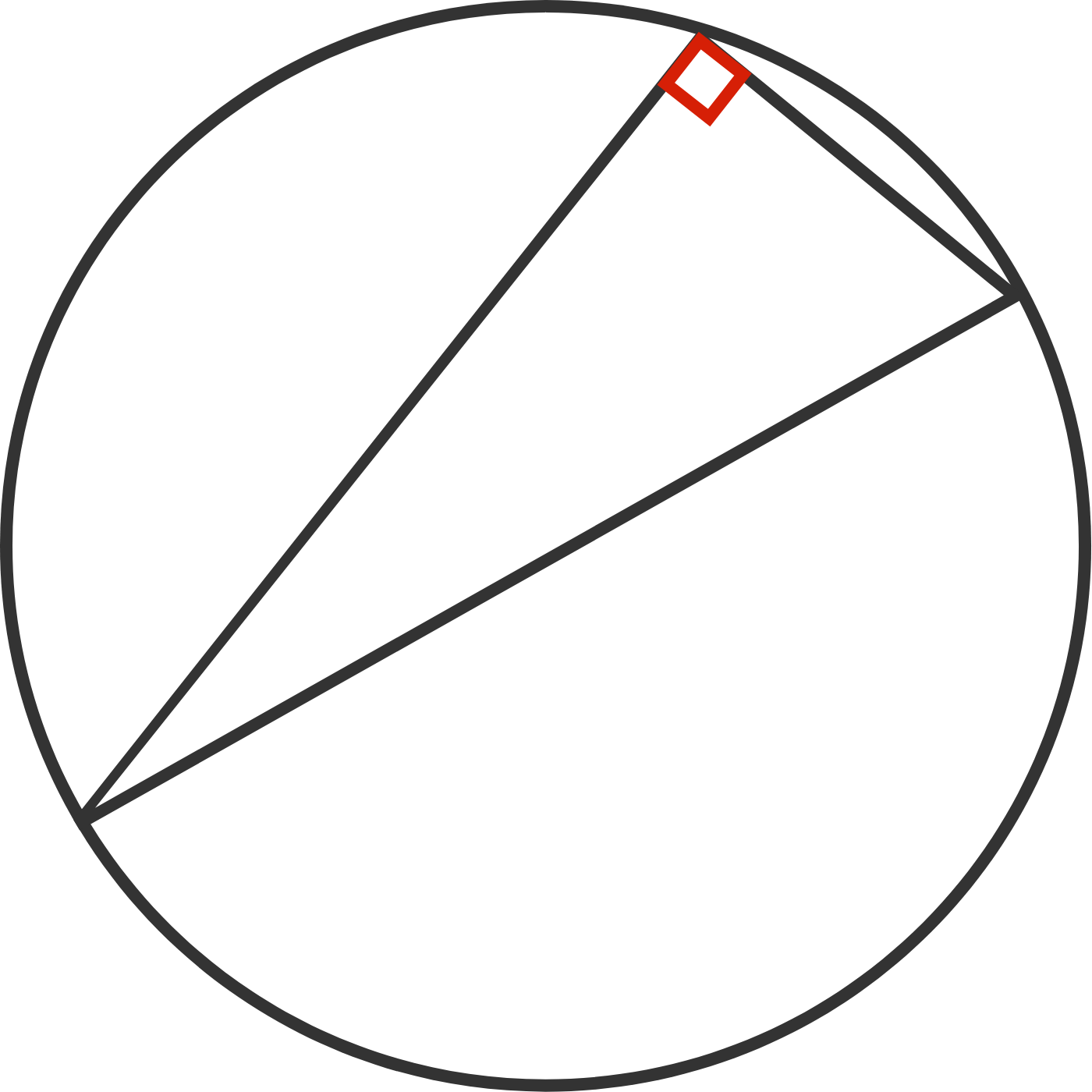Definition: A triangle is inscribed in a circle if all three of its corners lie on the circumference of the circle.

# Thales' Theorem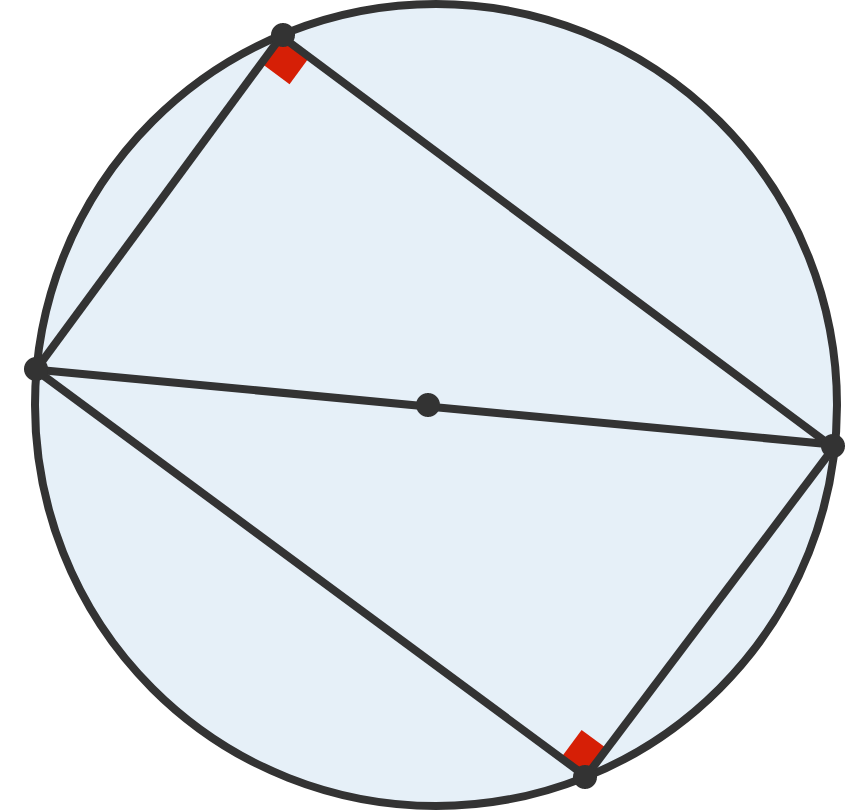The quadrilateral above is made by inscribing a right triangle in a circle, then rotating it $180^\circ$ and inscribing the triangle again as shown.

# Thales' Theorem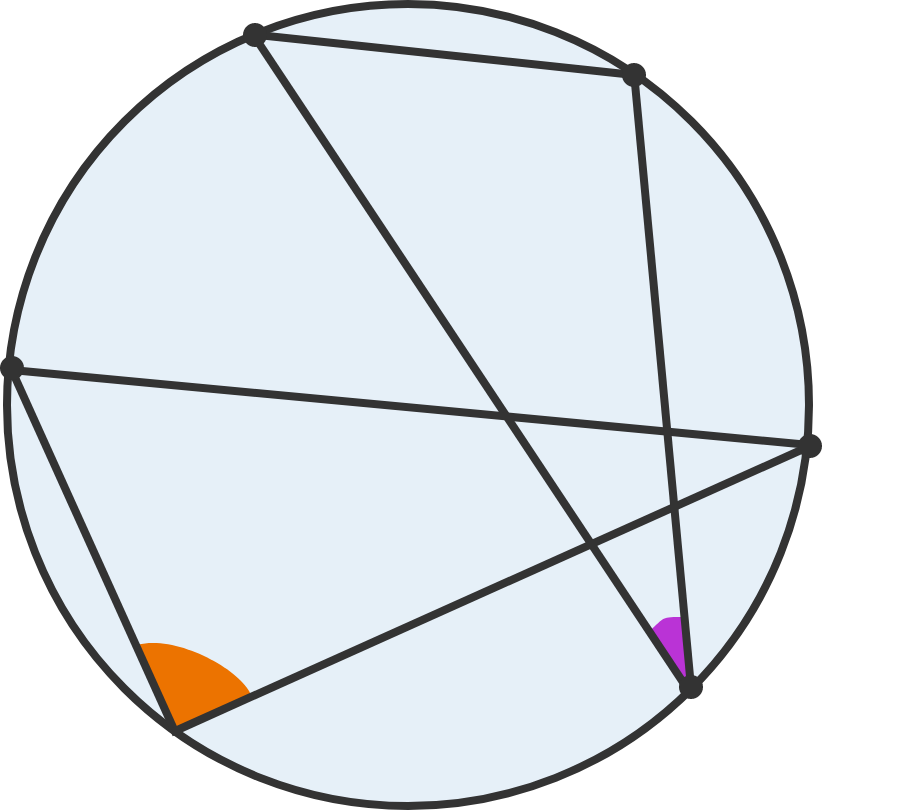Thales lets us know what happens if we inscribe an angle in a semicircle, but what if we just inscribe an angle in a circle in general? That will be the subject of the next quiz, and it's one of the most elegant theorems in geometry!

×